# Solving a Triangle: What Went Wrong?

Trigonometry can be a powerful tool for solving sides and angles in triangles. But you have to be careful with it! We’ll look at a classic type of error in solving an SSA triangle, get three explanations, and then see how knowing the context of a question can change our answer – to the point of skipping the trigonometry altogether!

## Why did I get the wrong angle?

The question came from Stoycho in early September:

Maybe the question is pretty stupid but I can’t figure out where is the problem?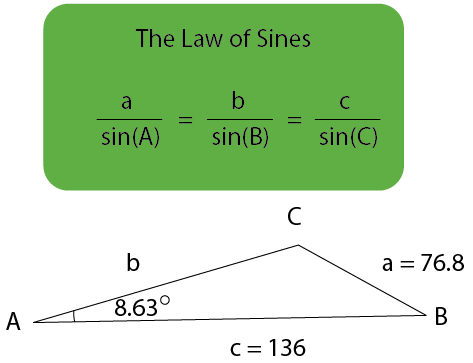So based on Law of Sines,

136 / sin(C) = 76.8 / sin(8.63°)

or

sin(C) = 136 / (76.8 / sin(8.63°)) = 0,2657189;
Angle C = 15,40967°

Obviously this is wrong, but where is the problem?

At this point, we don’t know the context of the problem; we’ll find out later. Given what we know, we can only assume this is an exercise in a trigonometry course. But we don’t have a full statement of the problem.

On the other hand, we can easily see what he is concerned about: Angle C looks obtuse in the picture; and although the picture does not have accurate angles, we can see that adjusting them would not change this fact. How did he get an acute angle for his answer?

### Two  angles with the same sine

Doctor Fenton was the first to answer:

The problem lies with taking the inverse sine of 136*sin(8.63)/76.8.  The range of the principal branch of the inverse sine function (usually written as Sin-1(x)) is the interval [-π/2, π/2], or angles in the fourth and first quadrant.  When you use a calculator (or tables) to evaluate θ = Sin-1(x) when x > 0, the result will always be a first quadrant angle.  You also need to consider the possibility that the angle you want is in the second quadrant, in which case the first quadrant solution θ1 will be the reference angle of the desired solution, or 180° – θ1.  Then the desired solution is θ = 180° – θ1.

If you have any questions, please write back and I will try to explain further.

Here is a demonstration of two such angles with the same sine, one in the first quadrant, the other in the second: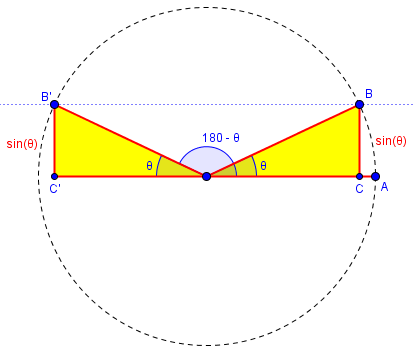On the unit circle, as shown, the sine of an angle is the y-coordinate of the point on the circle, and points B and B’ both have the same y-coordinate, so the sines of $$\theta$$ and $$180^\circ – \theta$$ are equal. So although $$\sin^{-1}(y)$$ is the first-quadrant angle $$\theta$$, the supplement of that angle is also a possible solution.

### The ambiguous case

Doctor Rick joined in, offering the bigger picture:

Hi, Stoycho. I would like to add that The Math Doctors has a pair of blogs on the broader topic raised here:

Solving an Oblique Triangle, Part I

Solving an Oblique Triangle, Part II

The first introduces the issue you’re confronting, in the section “The trouble with inverse sines”. The second goes into much more depth on the issue, “the ambiguous case”. That might be of help.

Also, let me point out that the solution you got is only “obviously wrong” based on the figure, in which angle C is “clearly” obtuse. But figures can’t be trusted; often on exams, there will be a statement to the effect that “figure is not to scale” – a reminder not to assume anything from the figure that isn’t clearly stated. Based solely on the given information (A = 8.63°, a = 76.8, c = 136), your solution is perfectly valid – but it is not the only solution. If the problem stated that C is obtuse, that would be a different story.

If the question was an exercise, it was improperly stated, as the picture implied that C was obtuse, but neither stated that nor asked for all solutions. As we’ll see, it is not an exercise – which reminds us that when we are solving a problem as part of a larger problem, we need to be careful to state the “subproblem” carefully, so as not to mislead ourselves.

### The full solution

Then I joined the party:

Here is an accurate picture showing both your triangle ABC, and the other valid solution, ABC’, which looks more like the given image (but shows that it is not to scale).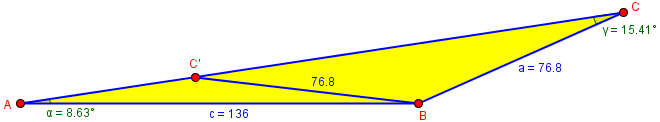Have you learned about the SSA case? If not, perhaps this exercise is intended to introduce the idea!

In other words, this is a “Side-Side-Angle problem” which typically has two solutions that look like this individually:

The triangle from Stoycho’s calculations …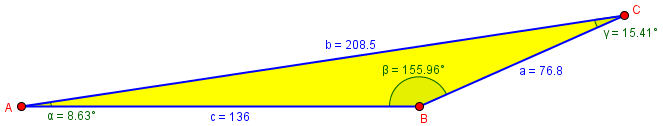… and what he really wanted, based on the picture: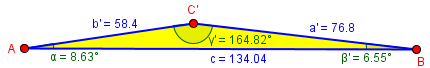Stoycho replied,

Thank you all for your help. I understand that depending on the type of triangle the formula considers the corresponding angle. My decision was correct but it just calculated the other angle.

## The real problem

But there was more to say, so I wrote back asking for context:

You haven’t shown us the entire problem, which is important. What did it ask you to do? Did it say anything more about the triangle?

I would expect it to have asked for all solutions; if it just said to solve the triangle, then that is implied (and includes finding both unknown angles and the unknown side).

And if so, then your answer is not complete. Finding one possibility for the angle does not finish the work. This is what all three of us were saying.

I had shown what the two solutions looked like, but not the actual solutions. Did he really need only the obtuse value of C, or something else?

Stoycho now showed us what he was really doing:

Thank you All. I attached image for what I ask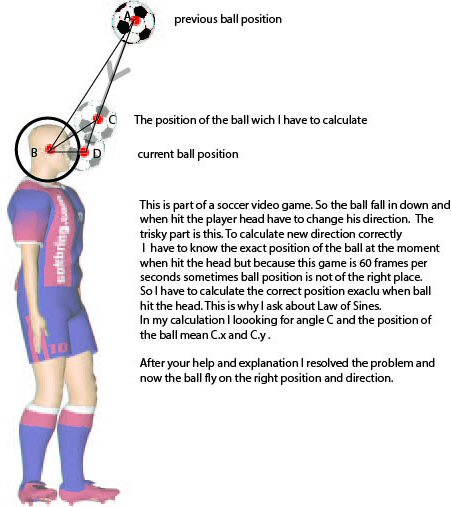This is a nice application of trigonometry. In computer simulation of a moving ball, we can just calculate its position at a sequence of times based on its velocity; but if something changes between frames (in this case, hitting the player’s head at point C, between successive calculated locations A and D), a calculation is needed to determine exactly when it hit, and at what angle, so that position D can be replaced with the appropriate location after the bounce. A game has to do a lot of work to produce the appearance of reality!

Here he isn’t looking for perfection; modeling how the ball would bounce off the irregular shape of a real head would be far harder than what he is doing, pretending the head is a perfect sphere! Presumably this looks real enough for a game. Distance BC is the sum of the radius of the imagined spherical head and the radius of the ball, which is the closest the center of the ball can get to the center of the head.

### An alternative method

Doctor Rick responded:

Hi, Stoycho.

Now we know that the problem was not assigned to you; it was of your own creation … so it was your job to amend the problem so as to indicate which of the two solutions to the original problem was the correct one in context. I assume you realized, after our discussions, that the additional condition is that angle ACB must be obtuse. The acute solution corresponds to a second time the ball intersects with the circle of radius BC, which is unphysical as the ball would have had to pass through the head to get there.

Until the possibility of two solutions was pointed out, he didn’t see the need to explicitly mention a requirement that angle C be obtuse (or, equivalently, that C is the nearer of the two possible points to A, the first place the ball’s center intersects the circle). This is common in problem-solving: As you try things, you discover new constraints.

If I had seen the actual problem at the start, I would have suggested an entirely different approach. Since you are evidently working with x and y coordinates, and you should know the coordinates of points A, B, and D, I would do the following: Write the equation of the line AD, and the equation of the circle of given radius centered at B. Then solve the system of these two equations.

You will again get two solutions (the line is a secant of the circle, intersecting it in two points). You might choose the solution whose x coordinate is closer to A’s x coordinate. Or you could compare y coordinates, or the squares of the distances of the two solutions from A. (This latter method avoids the need for a special case where AD is either vertical or horizontal.)

Let’s try this. I’ve put the picture on a grid (in GeoGebra) and found coordinates for the points: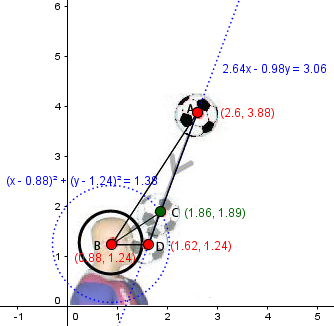The ball was at A$$(2.6,3.88)$$, and without the collision would now be at D$$(1.62,1.24)$$. We want to find where it intersects the circle centered at B$$(0.88,1.24)$$ with radius 1.18 (the sum of the radii of the “head” and the ball). We want to find the coordinates of C, which I will pretend I don’t know.

We find that the line AD has equation $$y=2.694x-3.122$$ and the circle has equation $$(x-0.88)^2+(y-1.24)^2=1.38$$

We can eliminate $$y$$ by substituting the line equation in the circle equation, obtaining $$(x-0.88)^2+(2.694x-4.362)^2=1.38$$

Expanding, this becomes $$8.257x^2-25.265x+18.325=0$$

By the quadratic formula, we have $$x=\frac{25.265\pm\sqrt{(-25.265)^2-4(8.257)(18.325)}}{2(8.257)}\\ = \frac{25.265\pm 5.451}{16.514} = 1.20\text{ or }1.86$$

The larger of these is closer to A, and is in fact the x-coordinate of C. The y-coordinate is $$y=2.694(1.86)-3.122=1.89$$

### The SSA method, applied

Let’s do the triangle calculations for this same figure: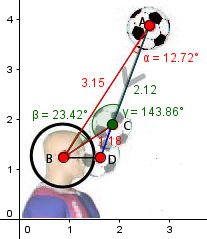The values in red are given: $$AB = 3.15$$, $$BC = 1.18$$, and $$\angle A = 12.72^\circ$$. By the Law of Sines, $$\frac{3.15}{\sin(C)}=\frac{1.18}{\sin(12.72^\circ)}$$ Solving for angle C, $$\sin(C)=\frac{3.15 \sin(12.72^\circ)}{1.18}=0.5878\\ C=\sin^{-1}(0.5878) = 36^\circ\text{ or }180-36=144^\circ$$ The picture shows a slightly different angle due to rounding. To locate point C, we can first find its distance from A: $$B=180-(12.72+144) = 23.28^\circ$$ $$\frac{b}{\sin(23.28^\circ)}=\frac{1.18}{\sin(12.72^\circ)}$$ $$b = \frac{1.18 \sin(23.28^\circ)}{\sin(12.72^\circ)} = 2.118$$ which is right.

Now we need to go that distance along line AD. To do that, we find vector AD to be $$\vec{AD} = \langle 1.62-2.6, 1.24-3.88\rangle = \langle -0.98, -2.64\rangle,$$ whose length is $$|\vec{AD}| = \sqrt{0.98^2+2.64^2}=2.816;$$ we need vector AC to be the appropriate scalar multiple of vector AD: $$\vec{AC} = \frac{2.118}{2.816}\vec{AD} = 0.752\vec{AD}$$ $$= \langle 0.752\cdot-0.98, 0.752\cdot-2.64\rangle = \langle -0.737, -1.986\rangle$$. Adding this to point A, we get $$(2.6-0.737, 3.88-1.986) = (1.863, 1.894)$$ which again agrees with the graph and the other method.

Which is better? That depends on the form in which the data are available. If points are stored as coordinates, then the coordinate method seems good. But if angles and distances are available, trig works well.

This site uses Akismet to reduce spam. Learn how your comment data is processed.